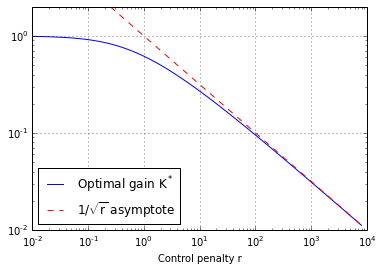# LQ control example¶

This Notebook illustrates the following libraries:

• symbolic math with SymPy (http://sympy.org)
• numerical regulator synthesis with python-control (http://python-control.sourceforge.net/manual/)
• (and a bit of plotting with matplotlib, http://matplotlib.org/)

...applied on the example of a Linear Quadratic control problem.

PH, December 2014

## 1) Compute the optimal LQ control symbolically¶

In :
import sympy
from sympy import symbols, simplify, Eq, solve

In :
sympy.init_printing()


integrator system, in discrete time:

$x_{k+1} = x_k + u_k$

In :
x, u = symbols('x_k u_k')
x_next = x+u
x_next

Out:
$$u_{k} + x_{k}$$

$c(x,u) = x^2 + r. u^2$

In :
r = symbols('r')
c = x**2 + r*u**2
c

Out:
$$r u_{k}^{2} + x_{k}^{2}$$

The optimal control is solution of Bellman equation:

$J(x) = \min_u c(x,u) + J(f(x,u))$

with $J(x)$ known to be quadratic: $J(x) = V x^2$

In :
V = symbols('V')
# left-hand side
Bell_lhs = V*x**2
# right-hand side
Bell_rhs = c+V*x_next**2
Bell_rhs

Out:
$$V \left(u_{k} + x_{k}\right)^{2} + r u_{k}^{2} + x_{k}^{2}$$

Minimize the right-hand side, by solving $d\cdot/du = 0$

In :
Bell_rhs.diff(u)

Out:
$$V \left(2 u_{k} + 2 x_{k}\right) + 2 r u_{k}$$
In :
solve(Bell_rhs.diff(u), u)

Out:
$$\begin{bmatrix}- \frac{V x_{k}}{V + r}\end{bmatrix}$$

The optimal control is a linear feedback:

$u^* = -K^* x$

In :
u_opt = solve(Bell_rhs.diff(u), u)
K_opt = -u_opt/x
K_opt

Out:
$$\frac{V}{V + r}$$

but, we still need to find $V$...

→ Reinject the optimal feedback in Bellman equation

In :
Bell_rhs_opt = Bell_rhs.subs(u, u_opt)
Bell_rhs_opt

Out:
$$\frac{V^{2} r x_{k}^{2}}{\left(V + r\right)^{2}} + V \left(- \frac{V x_{k}}{V + r} + x_{k}\right)^{2} + x_{k}^{2}$$

Solve for $V$ (Bellman eq. simplifies to a 2nd order polynomial root finding)

In :
solve(Bell_lhs-Bell_rhs_opt, V)

Out:
$$\begin{bmatrix}- \frac{1}{2} \sqrt{4 r + 1} + \frac{1}{2}, & \frac{1}{2} \sqrt{4 r + 1} + \frac{1}{2}\end{bmatrix}$$

And we know that $V>0$ and $r>0$, so we only keep the positive solution

In :
V_opt = solve(Bell_lhs-Bell_rhs_opt, V)
V_opt

Out:
$$\frac{1}{2} \sqrt{4 r + 1} + \frac{1}{2}$$

Final expression of the optimal gain:

In :
K_opt = simplify(K_opt.subs(V, V_opt))
K_opt

Out:
$$\frac{\sqrt{4 r + 1} + 1}{2 r + \sqrt{4 r + 1} + 1}$$

(notice: $K^*$ is equivalent to $\frac{1}{\sqrt{r}}$ when $r\to \infty$)

Special values of $K^*$:

In :
(K_opt.subs(r, 0),
K_opt.subs(r, 1).evalf(3),
K_opt.subs(r, 10).evalf(3))

Out:
$$\begin{pmatrix}1, & 0.618, & 0.27\end{pmatrix}$$

## 2) Plot the gain $K^*$, as a function of the control penalty $r$¶

Transform the symbolic expression in a numerical function, to be evaluated and plotted

In :
import numpy
from numpy import array, sqrt

In :
K_opt_r = sympy.lambdify(r, K_opt, numpy)

In :
K_opt_r(array([0, 1, 10]))

Out:
array([ 1.        ,  0.61803399,  0.27015621])

In :
import matplotlib.pyplot as plt
%matplotlib inline

In :
r_list = numpy.logspace(-2,3.9, 100)
plt.loglog(r_list, K_opt_r(r_list), label='Optimal gain $K^*$')
plt.loglog(r_list, 1/sqrt(r_list), 'r--', label='$1/\sqrt{r}$ asymptote')

plt.grid(True)
plt.xlabel('Control penalty r')
plt.ylim(ymax=2)
plt.legend(loc='lower left');## 3) Numerical control synthesis¶

Using python-control to find numerically the optimal feedback (http://python-control.sourceforge.net/manual/synthesis.html).

(Based on SLICOT Fortran routines http://slicot.org/ )

→ requires the control and slycot packages (and installing slycot requires a Fortran compiler, like gfortran on Linux, and lapack-dev for the lapack headers).

In :
import control


Linear quadratic regulator design with lqr (http://python-control.sourceforge.net/manual/synthesis.html#control.lqr)

problem: only for continous time. Discrete time not yet implemented (→ student project?).

In :
control.lqr??


Integrator, in continuous time:

$\dot{x} = u$

In :
A = 0
B = 1

Q = 1
R = 100

In :
K, S, E = control.lqr(A, B, Q, R)

In :
K

Out:
array([[ 0.1]])

In []:
1/numpy.sqrt(R)


### Recompute the optimal gain symbolically¶

In continuous time, the optimal control is solution of the Hamilton-Jacobi-Bellman (HJB) equation

$0 = \min_u(c(x,u) + \frac{\partial J}{\partial x} f(x,u))$

In :
x, u = symbols('x u')
x_der = u
x_der

Out:
$$u$$
In :
r = symbols('r')
c = x**2 + r*u**2
c

Out:
$$r u^{2} + x^{2}$$
In :
V = symbols('V')
J = V*x**2

HJB = c + J.diff(x)*x_der
HJB

Out:
$$2 V u x + r u^{2} + x^{2}$$

minimize with respect to $u$:

In :
solve(HJB.diff(u), u)

Out:
$$\begin{bmatrix}- \frac{V x}{r}\end{bmatrix}$$
In :
u_opt = solve(HJB.diff(u), u)
K_opt = -u_opt/x
K_opt

Out:
$$\frac{V}{r}$$

Solve for V

In :
solve(HJB.subs(u, u_opt), V)

Out:
$$\begin{bmatrix}- \sqrt{r}, & \sqrt{r}\end{bmatrix}$$
In :
V_opt = solve(HJB.subs(u, u_opt), V)
V_opt

Out:
$$\sqrt{r}$$

Optimal gain:

In :
K_opt = K_opt.subs(V, V_opt)
K_opt

Out:
$$\frac{1}{\sqrt{r}}$$

Observation: the optimal gains in discrete time and continuous time match for big values of $r$ (i.e. for systems with slow dynamics).